# GGPlot Examples Best Reference#### GGPlot Examples Best Reference

This article provides a gallery of ggplot examples, including: scatter plot, density plots and histograms, bar and line plots, error bars, box plots, violin plots and more.

Contents:

#### Related Book

GGPlot2 Essentials for Great Data Visualization in R

## Prerequisites

Load required packages and set the theme function theme_bw() as the default theme:

library(tidyverse)
library(ggpubr)
theme_set(
theme_bw() +
theme(legend.position = "top")
)

## Scatter plot

• Basic scatter plot with correlation coefficient. The function stat_cor() [ggpubr R package] is used to add the correlation coefficient.
library("ggpubr")
p <- ggplot(mtcars, aes(mpg, wt)) +
geom_point() +
geom_smooth(method = lm) +
stat_cor(method = "pearson", label.x = 20)
p• Contextual zoom. Key R function facet_zoom() [ggforce]
library(ggforce)
ggplot(iris, aes(Petal.Length, Petal.Width, colour = Species)) +
geom_point() +
facet_zoom(x = Species == "versicolor")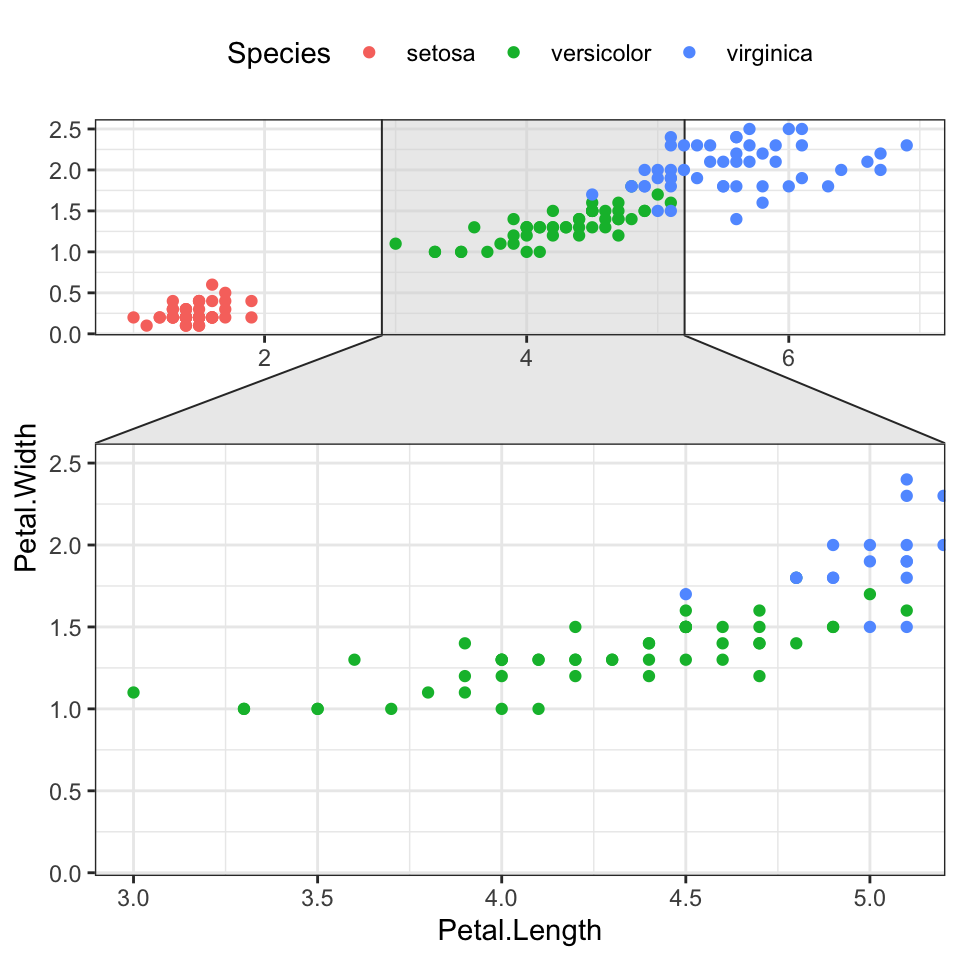• Encircle some points. The function geom_encircle() [ggalt R package] can be used to encircle a certain group of points
# Encircle setosa group
library("ggalt")
circle.df <- iris %>% filter(Species == "setosa")
ggplot(iris, aes(Petal.Length, Petal.Width)) +
geom_point(aes(colour = Species)) +
geom_encircle(data = circle.df, linetype = 2)• Create jittered points to avoid overlap. The overlapping points are randomly jittered around their original position based on a threshold controlled by the width argument in the function geom_jitter()
# Basic scatter plot
ggplot(mpg, aes(cty, hwy)) +
geom_point(size = 0.5)

# Jittered points
ggplot(mpg, aes(cty, hwy)) +
geom_jitter(size = 0.5, width = 0.5)• Create count charts to avoid overlap. Wherever there is more points overlap, the size of the circle gets bigger.
ggplot(mpg, aes(cty, hwy)) +
geom_count()• Bubble chart. In a bubble chart, points size is controlled by a continuous variable, here qsec.
ggplot(mtcars, aes(mpg, wt)) +
geom_point(aes(size = qsec), alpha = 0.5) +
scale_size(range = c(0.5, 12))  # Adjust the range of points size• Marginal density plots
library(ggpubr)
# Grouped Scatter plot with marginal density plots
ggscatterhist(
iris, x = "Sepal.Length", y = "Sepal.Width",
color = "Species", size = 3, alpha = 0.6,
palette = c("#00AFBB", "#E7B800", "#FC4E07"),
margin.params = list(fill = "Species", color = "black", size = 0.2)
)# Use box plot as marginal plots
ggscatterhist(
iris, x = "Sepal.Length", y = "Sepal.Width",
color = "Species", size = 3, alpha = 0.6,
palette = c("#00AFBB", "#E7B800", "#FC4E07"),
margin.plot = "boxplot",
ggtheme = theme_bw()
)## Distribution

### Density plot

• Basic density plot:
# Basic density plot
ggplot(iris, aes(Sepal.Length)) +
geom_density()

ggplot(iris, aes(Sepal.Length)) +
geom_density(fill = "lightgray") +
geom_vline(aes(xintercept = mean(Sepal.Length)), linetype = 2)• Change color by groups
# Change line color by groups
ggplot(iris, aes(Sepal.Length, color = Species)) +
geom_density() +
scale_color_viridis_d()# Add mean line by groups
mu <- iris %>%
group_by(Species) %>%
summarise(grp.mean = mean(Sepal.Length))

ggplot(iris, aes(Sepal.Length, color = Species)) +
geom_density() +
geom_vline(aes(xintercept = grp.mean, color = Species),
data = mu, linetype = 2) +
scale_color_viridis_d()### Histogram

• Basic histograms
# Basic histogram with mean line
ggplot(iris, aes(Sepal.Length)) +
geom_histogram(bins = 20, fill = "white", color = "black")  +
geom_vline(aes(xintercept = mean(Sepal.Length)), linetype = 2)

ggplot(iris, aes(Sepal.Length, stat(density))) +
geom_histogram(bins = 20, fill = "white", color = "black")  +
geom_density() +
geom_vline(aes(xintercept = mean(Sepal.Length)), linetype = 2)• Change color by groups
ggplot(iris, aes(Sepal.Length)) +
geom_histogram(aes(fill = Species, color = Species), bins = 20,
position = "identity", alpha = 0.5) +
scale_fill_viridis_d() +
scale_color_viridis_d()### QQ Plot

library(ggpubr)
ggqqplot(iris, x = "Sepal.Length",
ggtheme = theme_bw())### Empirical cumulative distribution (ECDF)

ggplot(iris, aes(Sepal.Length)) +
stat_ecdf(aes(color = Species)) +
scale_color_viridis_d()### Density ridgeline plots

The density ridgeline plot is an alternative to the standard geom_density() function that can be useful for visualizing changes in distributions, of a continuous variable, over time or space. Ridgeline plots are partially overlapping line plots that create the impression of a mountain range.

library(ggridges)
ggplot(iris, aes(x = Sepal.Length, y = Species)) +
geom_density_ridges(aes(fill = Species)) +
scale_fill_manual(values = c("#00AFBB", "#E7B800", "#FC4E07"))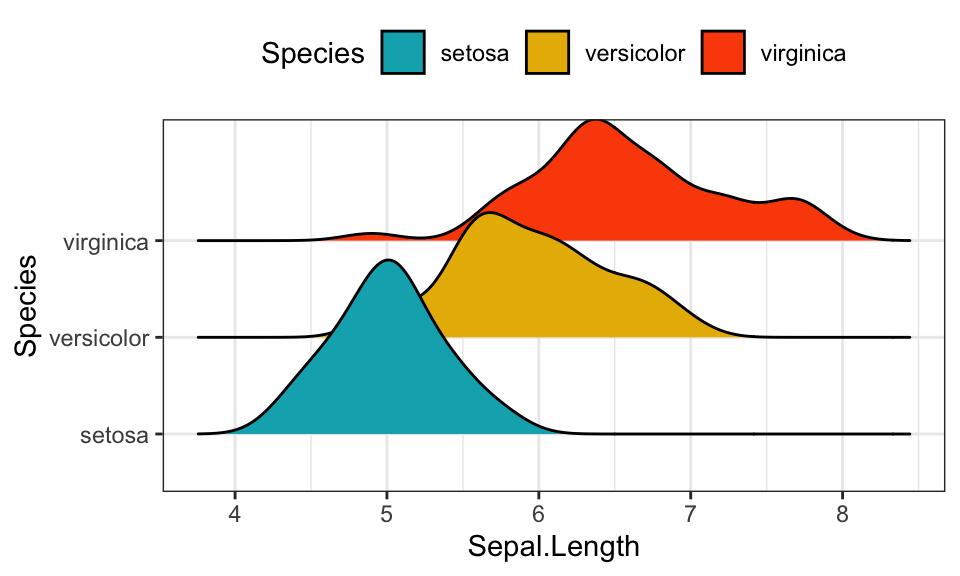## Bar charts and alternatives

• Data
df <- mtcars %>%
rownames_to_column() %>%
as_data_frame() %>%
mutate(cyl = as.factor(cyl)) %>%
select(rowname, wt, mpg, cyl)
df
## # A tibble: 32 x 4
##   rowname              wt   mpg cyl
##   <chr>             <dbl> <dbl> <fct>
## 1 Mazda RX4          2.62  21   6
## 2 Mazda RX4 Wag      2.88  21   6
## 3 Datsun 710         2.32  22.8 4
## 4 Hornet 4 Drive     3.22  21.4 6
## 5 Hornet Sportabout  3.44  18.7 8
## 6 Valiant            3.46  18.1 6
## # ... with 26 more rows
• Basic bar plots
# Basic bar plots
ggplot(df, aes(x = rowname, y = mpg)) +
geom_col() +
rotate_x_text(angle = 45)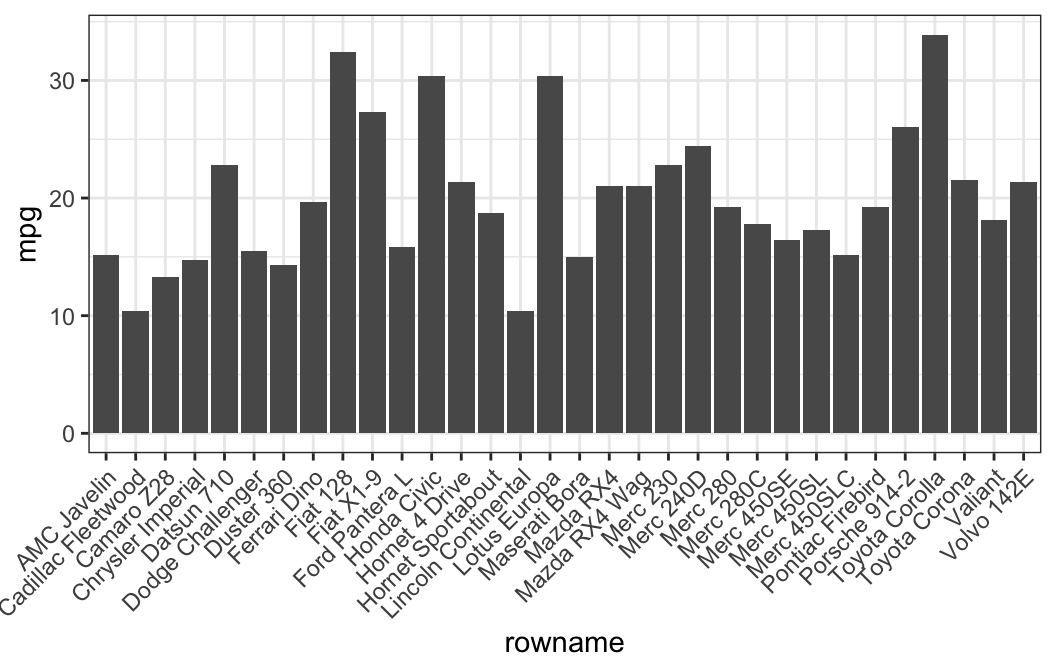# Reorder row names by mpg values
ggplot(df, aes(x = reorder(rowname, mpg), y = mpg)) +
geom_col()  +
rotate_x_text(angle = 45)• Horizontal bar plots
# Horizontal bar plots,
# change fill color by groups and add text labels
ggplot(df, aes(x = reorder(rowname, mpg), y = mpg)) +
geom_col( aes(fill = cyl)) +
geom_text(aes(label = mpg), nudge_y = 2) +
coord_flip() +
scale_fill_viridis_d()• Order bars by groups and by mpg values
df2 <- df %>%
arrange(cyl, mpg) %>%
mutate(rowname = factor(rowname, levels = rowname))

ggplot(df2, aes(x = rowname, y = mpg)) +
geom_col( aes(fill = cyl)) +
scale_fill_viridis_d() +
rotate_x_text(45)• Lollipop chart: Lollipop is an alternative to bar charts when you have large data sets.
ggplot(df2, aes(x = rowname, y = mpg)) +
geom_segment(
aes(x = rowname, xend = rowname, y = 0, yend = mpg),
color = "lightgray"
) +
geom_point(aes(color = cyl), size = 3) +
scale_color_viridis_d() +
theme_pubclean() +
rotate_x_text(45)• Bar plot with multiple groups
# Data
df3 <- data.frame(supp=rep(c("VC", "OJ"), each=3),
dose=rep(c("D0.5", "D1", "D2"),2),
len=c(6.8, 15, 33, 4.2, 10, 29.5))

# Stacked bar plots of y = counts by x = cut,
# colored by the variable color
ggplot(df3, aes(x = dose, y = len)) +
geom_col(aes(color = supp, fill = supp), position = position_stack()) +
scale_color_manual(values = c("#0073C2FF", "#EFC000FF"))+
scale_fill_manual(values = c("#0073C2FF", "#EFC000FF"))

# Use position = position_dodge()
ggplot(df3, aes(x = dose, y = len)) +
geom_col(aes(color = supp, fill = supp), position = position_dodge(0.8), width = 0.7) +
scale_color_manual(values = c("#0073C2FF", "#EFC000FF"))+
scale_fill_manual(values = c("#0073C2FF", "#EFC000FF"))## Line plot

# Data
df3 <- data.frame(supp=rep(c("VC", "OJ"), each=3),
dose=rep(c("D0.5", "D1", "D2"),2),
len=c(6.8, 15, 33, 4.2, 10, 29.5))

# Line plot
ggplot(df3, aes(x = dose, y = len, group = supp)) +
geom_line(aes(linetype = supp)) +
geom_point(aes(shape = supp))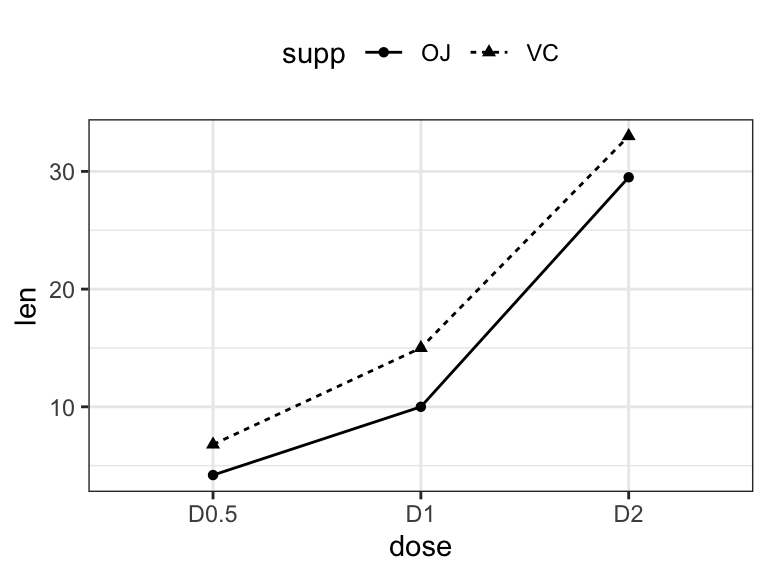## Error bars

• Data
# Raw data
df <- ToothGrowth %>% mutate(dose = as.factor(dose))
head(df, 3)
##    len supp dose
## 1  4.2   VC  0.5
## 2 11.5   VC  0.5
## 3  7.3   VC  0.5
# Summary statistics
df.summary <- df %>%
group_by(dose) %>%
summarise(sd = sd(len, na.rm = TRUE), len = mean(len))
df.summary
## # A tibble: 3 x 3
##   dose     sd   len
##   <fct> <dbl> <dbl>
## 1 0.5    4.50  10.6
## 2 1      4.42  19.7
## 3 2      3.77  26.1
• Basic line and bar plots with error bars
# (1) Line plot
ggplot(df.summary, aes(dose, len)) +
geom_line(aes(group = 1)) +
geom_errorbar( aes(ymin = len-sd, ymax = len+sd),width = 0.2) +
geom_point(size = 2)

# (2) Bar plot
ggplot(df.summary, aes(dose, len)) +
geom_bar(stat = "identity", fill = "lightgray", color = "black") +
geom_errorbar(aes(ymin = len, ymax = len+sd), width = 0.2)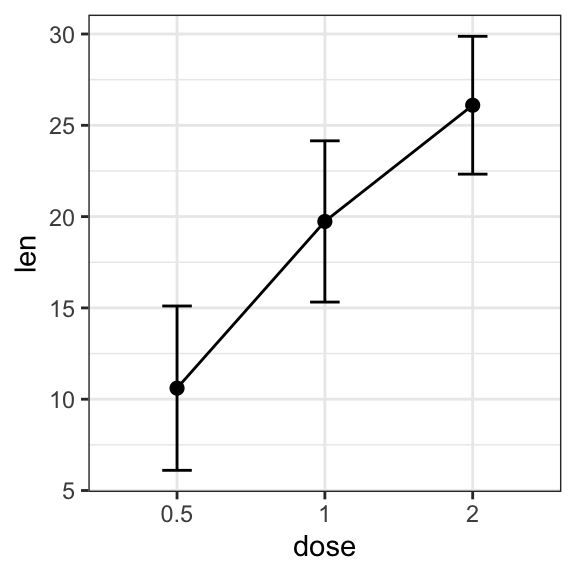• Grouped line/bar plots
# Data preparation
df.summary2 <- df %>%
group_by(dose, supp) %>%
summarise( sd = sd(len), len = mean(len))
df.summary2
## # A tibble: 6 x 4
## # Groups:   dose [?]
##   dose  supp     sd   len
##   <fct> <fct> <dbl> <dbl>
## 1 0.5   OJ     4.46 13.2
## 2 0.5   VC     2.75  7.98
## 3 1     OJ     3.91 22.7
## 4 1     VC     2.52 16.8
## 5 2     OJ     2.66 26.1
## 6 2     VC     4.80 26.1
# (1) Line plot + error bars
ggplot(df.summary2, aes(dose, len)) +
geom_line(aes(linetype = supp, group = supp))+
geom_point()+
geom_errorbar(
aes(ymin = len-sd, ymax = len+sd, group = supp),
width = 0.2
)# (2) Bar plots + upper error bars.
ggplot(df.summary2, aes(dose, len)) +
geom_bar(aes(fill = supp), stat = "identity",
position = position_dodge(0.8), width = 0.7)+
geom_errorbar(
aes(ymin = len, ymax = len+sd, group = supp),
width = 0.2, position = position_dodge(0.8)
)+
scale_fill_manual(values = c("grey80", "grey30"))## Box plots and alternatives

• Data
ToothGrowth$dose <- as.factor(ToothGrowth$dose)
• Basic box plots
# Basic
ggplot(ToothGrowth, aes(dose, len)) +
geom_boxplot()

# Box plot + violin plot
ggplot(ToothGrowth, aes(dose, len)) +
geom_violin(trim = FALSE) +
geom_boxplot(width = 0.2)• Add jittered points and dot plot
# Add jittered points
ggplot(ToothGrowth, aes(dose, len)) +
geom_boxplot() +
geom_jitter(width = 0.2)

# Dot plot + box plot
ggplot(ToothGrowth, aes(dose, len)) +
geom_boxplot() +
geom_dotplot(binaxis = "y", stackdir = "center")• Grouped plots
# Box plots
ggplot(ToothGrowth, aes(dose, len)) +
geom_boxplot(aes(color = supp)) +
scale_color_viridis_d()

ggplot(ToothGrowth, aes(dose, len, color = supp)) +
geom_boxplot() +
geom_jitter(position = position_jitterdodge(jitter.width = 0.2)) +
scale_color_viridis_d()## Time series data visualization

# Data preparation
df <- economics %>%
select(date, psavert, uempmed) %>%
gather(key = "variable", value = "value", -date)
head(df, 3)
## # A tibble: 3 x 3
##   date       variable value
##   <date>     <chr>    <dbl>
## 1 1967-07-01 psavert   12.5
## 2 1967-08-01 psavert   12.5
## 3 1967-09-01 psavert   11.7
# Multiple line plot
ggplot(df, aes(x = date, y = value)) +
geom_line(aes(color = variable), size = 1) +
scale_color_manual(values = c("#00AFBB", "#E7B800")) +
theme_minimal()## scatter plot matrix

library(GGally)
ggpairs(iris[,-5])+ theme_bw()## Correlation analysis

library("ggcorrplot")
# Compute a correlation matrix
my_data <- mtcars[, c(1,3,4,5,6,7)]
corr <- round(cor(my_data), 1)
# Visualize
ggcorrplot(corr, p.mat = cor_pmat(my_data),
hc.order = TRUE, type = "lower",
color = c("#FC4E07", "white", "#00AFBB"),
outline.col = "white", lab = TRUE)## Cluster analysis

library(factoextra)
USArrests %>%
scale() %>%                           # Scale the data
dist() %>%                            # Compute distance matrix
hclust(method = "ward.D2") %>%        # Hierarchical clustering
fviz_dend(cex = 0.5, k = 4, palette = "jco") # Visualize and cut
# into 4 groups## Balloon plot

Balloon plot is an alternative to bar plot for visualizing a large categorical data.

library(ggpubr)
# Data preparation
row.names = 1
)
head(housetasks, 4)
##            Wife Alternating Husband Jointly
## Laundry     156          14       2       4
## Main_meal   124          20       5       4
## Dinner       77          11       7      13
## Breakfeast   82          36      15       7
# Visualization
scale_fill_viridis_c(option = "C")•SFer

Kassambara
– thanks for this great reference!.

Q:
In the 1st example,
what would the code be
to print (as top legend):
R and R2 and p ?
(right now, the ex. only shows R and P…).

Thanks,
SFer

•Sfer

Kassambara
– thanks for this great reference!.

Q:
In the 1st example,
what would the code be
to print (as top legend):
R and R2 and P ?
(right now, the ex. only shows: R and P…).

Thanks,
SFer
San Francisco

•Kassambara

Please try the following R code:

library("ggpubr")
ggplot(mtcars, aes(mpg, wt)) +
geom_point() +
geom_smooth(method = lm) +
stat_cor(
aes(label = paste(..r.label.., ..rr.label.., ..p.label.., sep = "~,~")),
method = "pearson", label.x = 20
)
`

The output should look like this: